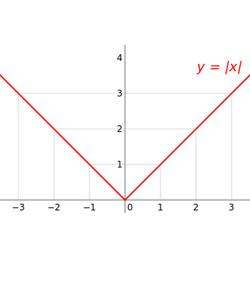# Module Quiz - Absolute Value And Integers

8 Questions | Attempts: 482
ShareSettingsThis is a quiz that includes absolute value and addition of integers.   All questions are multiple choice and only have one correct answer.   You can take the quiz as many times as necessary until you have a score of at least 80%.   To start the quiz, type in your full name (first name and last name) and click on "START".

• 1.
Choose the correct answer. l -4 l = ?
• A.

4

• B.

-4

• C.

0

• 2.
Choose the correct answer. l 4 l = ?
• A.

4

• B.

-4

• C.

0

• 3.
Add the integers.  Choose the correct answer. ( - 5 ) + ( -2 )
• A.

-7

• B.

7

• C.

-3

• D.

3

• 4.
• A.

-7

• B.

7

• C.

-3

• D.

3

• 5.
Add the integers.  Choose the correct answer. ( - 3 )  +  2
• A.

-1

• B.

1

• C.

-5

• D.

5

• 6.
Add the integers.  Choose the correct answer. 3  + ( -2 )
• A.

-1

• B.

1

• C.

-5

• D.

5

• 7.
Add the integers.  Choose the correct answer. ( - 10 )  +  3
• A.

-7

• B.

7

• C.

-13

• D.

13

• 8.
Add the integers.  Choose the correct answer. 8  + ( -5 )
• A.

-3

• B.

3

• C.

-13

• D.

13

## Related TopicsBack to top
×

Wait!
Here's an interesting quiz for you.